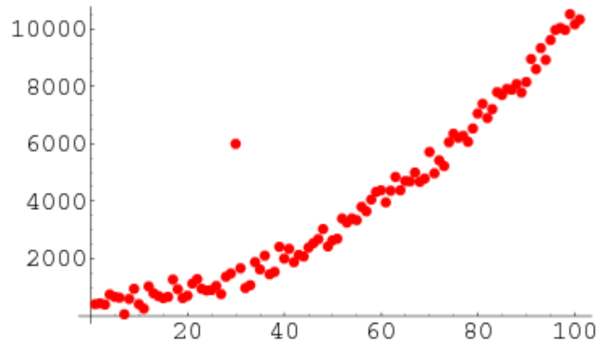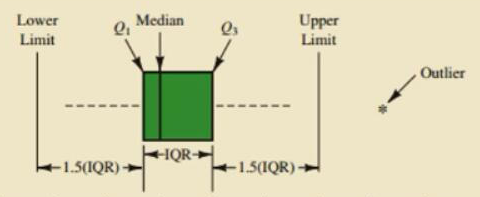# 数据分析 第五篇：异常值分析## 一，离群点检测

1，Z-score方法

3σ原则

Z-score = (Observation — Mean)/Standard Deviation
z = (X — μ) / σ

Z-score需要根据经验和实际情况来决定，通常把远离标准差3倍距离以上的数据点视为离群点，也就是说，把Z-score大于3的数据点视作离群点，Python代码的实现如下：

import numpy as np
import pandas as pd

def detect_outliers(data,threshold=3):
mean_d = np.mean(data)
std_d = np.std(data)
outliers = []

for y in data_d:
z_score= (y - mean_d)/std_d
if np.abs(z_score) > threshold:
outliers.append(y)
return outliers

2，IQR方法

IQR = Q3 − Q1

IQR是统计分散程度的一个度量，分散程度通过需要借助箱线图来观察，通常把小于 Q1 - 1.5 * IQR 或者大于 Q3 + 1.5 * IQR的数据点视作离群点，探测离群点的公式是：

outliers =  value < ( Q1 - 1.5 * IQR )  or value > ( Q3 + 1.5 * IQR )• 中心位置：中位数所在的位置就是数据集的中心，从中心位置向上或向下看，可以看出数据的倾斜程度。
• 散布程度：箱线图分为多个区间，区间较短时，表示落在该区间的点较集中；
• 对称性：如果中位数位于箱子的中间位置，那么数据分布较为对称；如果极值离中位数的距离较大，那么表示数据分布倾斜。
• 离群点：离群点分布在箱线图的上下边缘之外。

def detect_outliers(sr):
q1 = sr.quantile(0.25)
q3 = sr.quantile(0.75)
iqr = q3-q1 #Interquartile range
fence_low  = q1-1.5*iqr
fence_high = q3+1.5*iqr
outliers = sr.loc[(sr < fence_low) | (sr > fence_high)]
return outliers

## 二，异常值的处理

• 删除含有异常值的记录；
• 插补，把异常值视为缺失值，使用缺失值的处理方法进行处理，好处是利用现有数据对异常值进行替换，或插补；
• 不处理，直接在含有异常值的数据集上进行数据分析；

posted @ 2019-12-27 18:53  悦光阴  阅读(8041)  评论(0编辑  收藏  举报